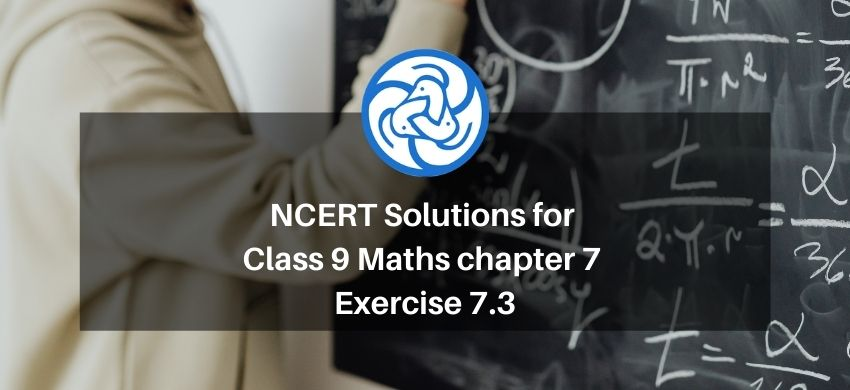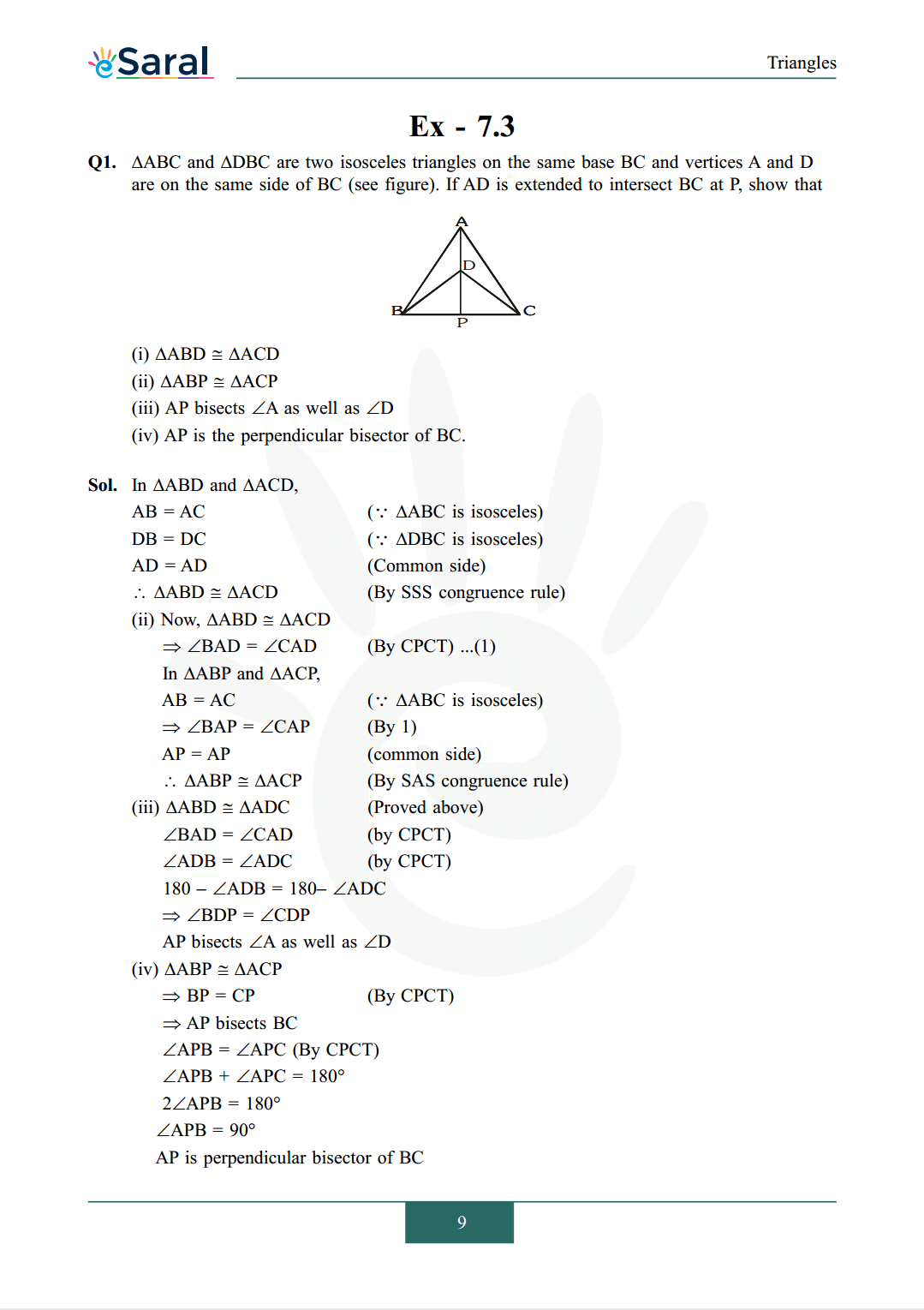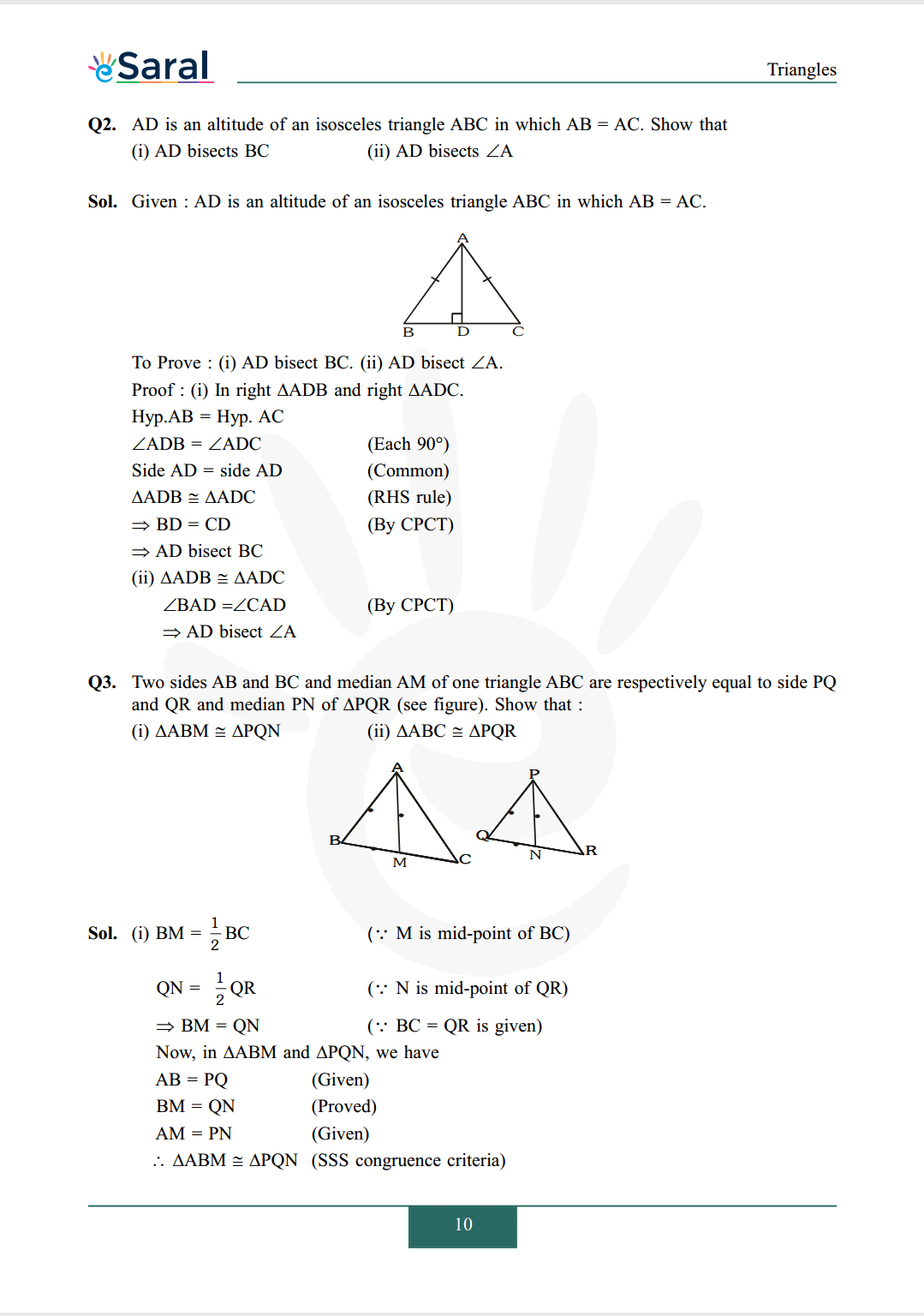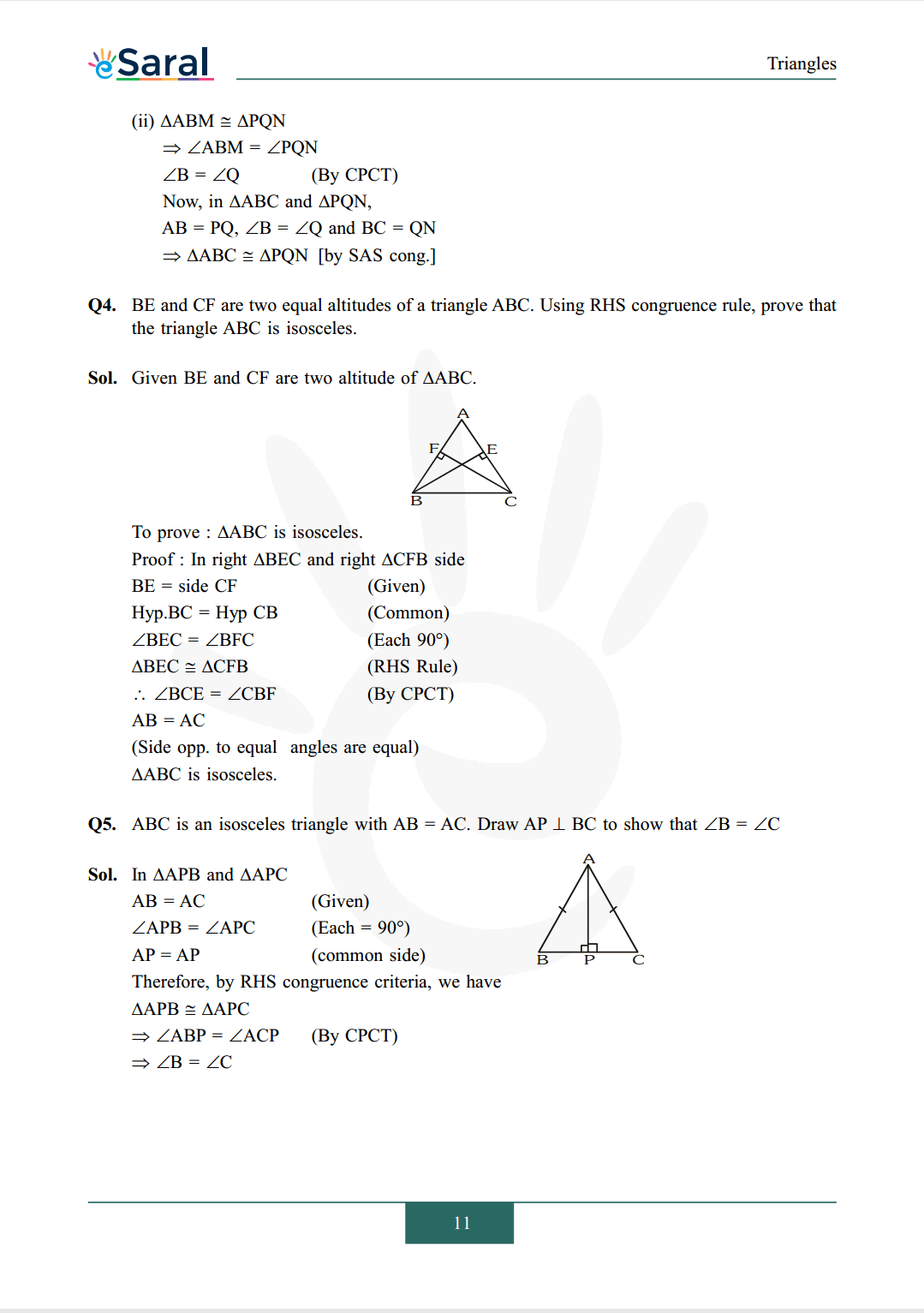Most Affordable JEE | NEET | 8,9,10 Preparation by Kota's Top IITian Doctor Faculties

# NCERT Solutions for Class 9 Maths chapter 7 Exercise 7.3 - Triangles`
Hey, are you a class 9 Student and Looking for Ways to Download NCERT Solutions for Class 9 Maths chapter 7 Exercise 7.3? If Yes then you are at the right place.

Here we have listed Class 9 maths chapter 7 exercise 7.3 solutions in PDF that is prepared by Kota’s top IITian’s Faculties by keeping Simplicity in mind.

If you want to score high in your class 9 Maths Exam then it is very important for you to have a good knowledge of all the important topics, so to learn and practice those topics you can use eSaral NCERT Solutions.

So, without wasting more time Let’s start.

### Download The PDF of NCERT Solutions for Class 9 Maths chapter 7 Exercise 7.3 "Triangles"#### All Questions of Chapter 7 Exercise 7.3

Once you complete the chapter 7 then you can revise Ex. 7.3 by solving following questions

Q1. $\triangle \mathrm{ABC}$ and $\Delta \mathrm{DBC}$ are two isosceles triangles on the same base $\mathrm{BC}$ and vertices $\mathrm{A}$ and $\mathrm{D}$ are on the same side of $\mathrm{BC}$ (see figure). If $\mathrm{AD}$ is extended to intersect $\mathrm{BC}$ at $\mathrm{P}$, show that(i) $\triangle \mathrm{ABD} \cong \triangle \mathrm{ACD}$
(ii) $\triangle \mathrm{ABP} \cong \triangle \mathrm{ACP}$
(iii) AP bisects $\angle \mathrm{A}$ as well as $\angle \mathrm{D}$
(iv) AP is the perpendicular bisector of $\mathrm{BC}$.

Q2. $\mathrm{AD}$ is an altitude of an isosceles triangle $\mathrm{ABC}$ in which $\mathrm{AB}=\mathrm{AC}$. Show that
(i) AD bisects $\mathrm{BC}$
(ii) AD bisects $\angle \mathrm{A}$

Q3. Two sides $\mathrm{AB}$ and $\mathrm{BC}$ and median $\mathrm{AM}$ of one triangle $\mathrm{ABC}$ are respectively equal to side $\mathrm{PQ}$ and QR and median PN of $\Delta \mathrm{PQR}$ (see figure). Show that :
(i) $\Delta \mathrm{ABM} \cong \Delta \mathrm{PQN}$
(ii) $\Delta \mathrm{ABC} \cong \Delta \mathrm{PQR}$Q4. BE and CF are two equal altitudes of a triangle ABC. Using RHS congruence rule, prove that the triangle ABC is isosceles.

Q5. $\mathrm{ABC}$ is an isosceles triangle with $\mathrm{AB}=\mathrm{AC}$. Draw $\mathrm{AP} \perp \mathrm{BC}$ to show that $\angle \mathrm{B}=\angle \mathrm{C}$

Class 9 NCERT Maths Book Chapterwise PDF

Class 9 NCERT Maths Exemplar Chapterwise PDF

Complete Solutions for Class 9 chapter 7

Class 9 Maths Chapter 6 Exercise 6.1 Solutions Free

Class 9 Maths Chapter 6 Exercise 6.2 Solutions Free

Class 9 Maths Chapter 6 Exercise 6.3 Solutions Free

If you have any Confusion related to NCERT Solutions for Class 9 Maths chapter 7 Exercise 7.3 then feel free to ask in the comments section down below.

To watch Free Learning Videos on Class 9 by Kota’s top Faculties Install the eSaral App